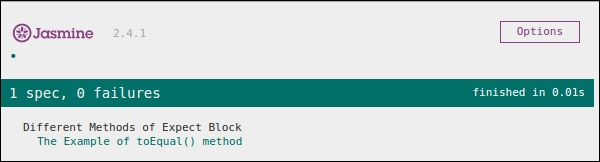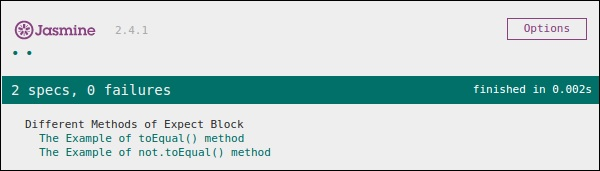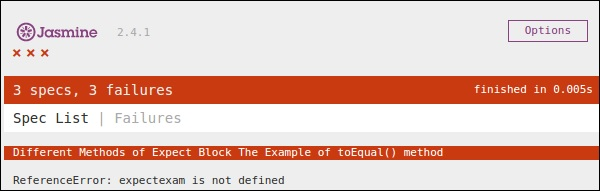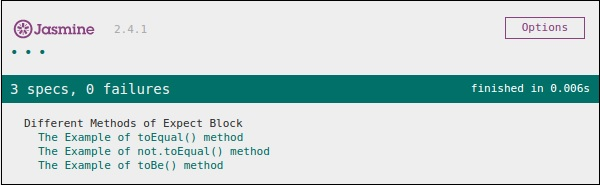# JasmineJS - Equality Check

Jasmine provides plenty of methods which help us check the equality of any JavaScript function and file. Following are some examples to check equality conditions.

## ToEqual()

ToEqual() is the simplest matcher present in the inbuilt library of Jasmine. It just matches whether the result of the operation given as an argument to this method matches with the result of it or not.

The following example will help you understand how this matcher works. We have two files to be tested named as “expectexam.js” and another one through which we need to test is “expectSpec.js”.

### Expectexam.js

```window.expectexam = {
currentVal: 0,
};
```

### ExpectSpec.js

```describe("Different Methods of Expect Block",function () {

it("The Example of toEqual() method",function () {
//this will check whether the value of the variable
// currentVal is equal to 0 or not.
expect(expectexam.currentVal).toEqual(0);
});
});
```

On successful execution, these pieces of code will yield the following output. Remember you need to add these files into the header section of specRunner.html file as directed in the earlier example.## not.toEqual()

not.toEqual() works exactly opposite to toEqual(). not.toEqual() is used when we need to check if the value does not match with the output of any function.

We will modify the above example to show how this works.

### ExpectSpec.js

```describe("Different Methods of Expect Block",function () {

it("The Example of toEqual() method",function () {
expect(expectexam.currentVal).toEqual(0);
});

it("The Example of not.toEqual() method",function () {
//negation  testing expect(expectexam.currentVal).not.toEqual(5);
});
});
```

### Expectexam.js

```window.expectexam = {
currentVal: 0,
};
```

In the second expect block, we are checking whether the value of the currentVal is equal to 5 as the value of currentVal is zero hence our test passes and provides us with a green output.## ToBe()

toBe() matcher works in a similar way as toEqual(), however they are technically different from each other. toBe() matcher matches with the type of the object whereas toEqual() matches with the equivalency of the result.

The following example will help you understand the working principle of the toBe() matcher. This matcher is exactly equivalent to the “===” operator of JavaScript whereas toEqual() is similar to the “==” operator of JavaScript.

### ExpectSpec.js

```describe("Different Methods of Expect Block",function () {

it("The Example of toBe() method",function () {
expect(expectexam.name).toBe(expectexam.name1);
});
});
```

### Expectexam.js

```window.expectexam = {
currentVal: 0,
name:"tutorialspoint",
name1:tutorialspoint
};
```

We will slightly modify our expectexam JavaScript file. We added two new variables, name and name1. Please find the difference between these two added variables - one is of string type and another one is not a string type.

Following screenshot is our test result where the red cross depicts that these two values are not equal, whereas it is expected to be equal. Hence our test fails.Let us turn both the variables, name and name1 as String type variables and run the same SpecRunner.html again. Now check the output. It will prove that toBe() not only matches with the equivalency of the variable, but it also matches with the data type or object type of the variable.

## not.toBe()

As seen earlier, not is nothing but a negation of the toBe() method. It fails when the expected result matches with the actual output of the function or JavaScript file.

Following is a simple example that will help you understand how not.toBe() matcher works.

```describe("Different Methods of Expect Block",function () {
it("The Example of not.toBe() method",function () {
expect(true).not.toBe(false);
});
});
```

Here Jasmine will try to match up true with false. As true cannot be same as false, this test case will be valid and pass through.